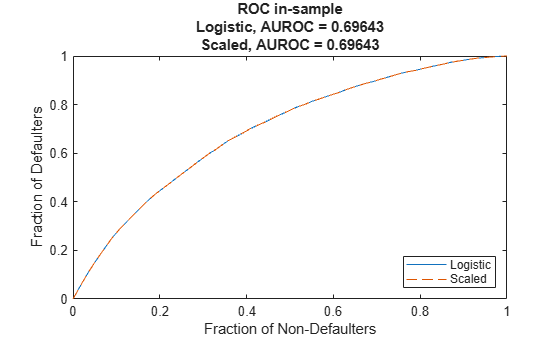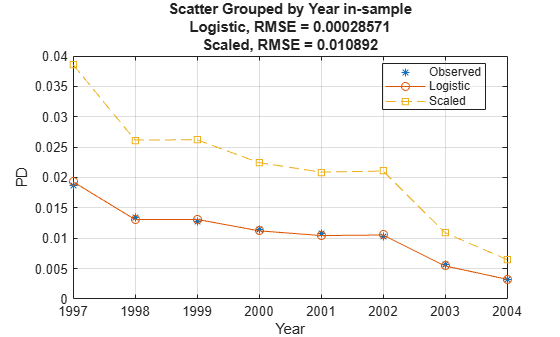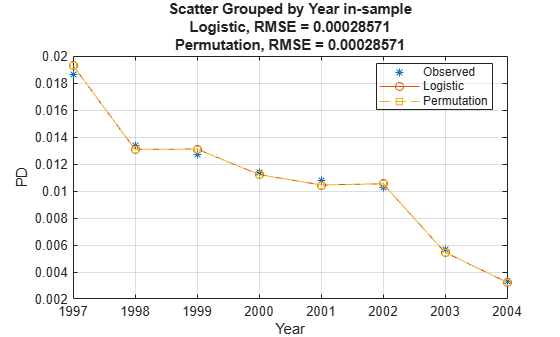Compare Model Discrimination and Accuracy to Validate of Probability of Default

This example shows some differences between discrimination and accuracy metrics for the validation of probability of default (PD) models.

The lifetime PD models in Risk Management Toolbox™ (see fitLifetimePDModel) support the area under the receiver operating characteristic curve (AUROC) as a discrimination (rank-ordering performance) metric and the root mean squared error (RMSE) as an accuracy (calibration) metric. The AUROC metric measures ranking, whereas the RMSE measures the precision of the predicted values. The example shows that it is possible to have:

• Same discrimination, different accuracy

• Same accuracy, different discrimination

Therefore, it is important to look at both discrimination and accuracy as part of a model validation framework.

There are several different metrics for PD model discrimination and model accuracy. For more information, see References. Different metrics may have different characteristics and the behavior demonstrated in this example does not necessarily generalize to other discrimination and accuracy metrics. The goal of this example is to emphasize the importance of using both discrimination and accuracy metrics to assess model predictions.

data = join(data,dataMacro);
'AgeVar','YOB',...
'IDVar','ID',...
'LoanVars','ScoreGroup',...
'MacroVars',{'GDP','Market'},...
'ResponseVar','Default');
disp(pdModel)
Logistic with properties:

ModelID: "Logistic"
Description: ""
Model: [1x1 classreg.regr.CompactGeneralizedLinearModel]
IDVar: "ID"
AgeVar: "YOB"
LoanVars: "ScoreGroup"
MacroVars: ["GDP"    "Market"]
ResponseVar: "Default"

Same Discrimination, Different Accuracy

Discrimination measures only ranking of customers, that is, whether riskier customers get assigned higher PDs than less risky customers. Therefore, if you scale the probabilities or apply another monotonic transformation that results in valid probabilities, the AUROC measure does not change.

For example, multiply the predicted PDs by a factor of 2, which preserves the ranking (where the worse customers have higher PDs). To compare the results, pass the modified PDs as reference PDs.

PD0 = predict(pdModel,data);
PD1 = 2*PD0;

disp([PD0(1:10) PD1(1:10)])
0.0090    0.0181
0.0052    0.0104
0.0044    0.0088
0.0038    0.0076
0.0035    0.0071
0.0036    0.0072
0.0019    0.0037
0.0011    0.0022
0.0164    0.0328
0.0094    0.0189

Verify that the discrimination measure is not affected using modelDiscriminationPlot.

modelDiscriminationPlot(pdModel,data,'DataID','in-sample','ReferencePD',PD1,'ReferenceID','Scaled')Use modelAccuracyPlot to visualize the observed default rates compared to the predicted probabilities of default (PD). The accuracy, however, is severely affected by the change. The modified PDs are far away from the observed default rates and the RMSE for the modified PDs is orders of magnitude higher than the RMSE of the original PDs.

modelAccuracyPlot(pdModel,data,'Year',"DataID",'in-sample','ReferencePD',PD1,"ReferenceID",'Scaled')Same Accuracy, Different Discrimination

On the other hand, you can also modify the predicted PDs to keep the accuracy metric unchanged and worsen the discrimination metric.

One way to do this is to permute the PDs within a group. By doing this, the ranking within each group is affected, but the average PD for the group is unchanged.

rng('default'); % for reproducibility
PD1 = PD0;
for Year=1997:2004
Ind = data.Year==Year;
PDYear = PD0(Ind);
PD1(Ind) = PDYear(randperm(length(PDYear)));
end

Verify that the discrimination measure is worse for the modified PDs using modelDiscriminationPlot.

modelDiscriminationPlot(pdModel,data,'DataID','in-sample','ReferencePD',PD1,'ReferenceID','Permutation')The modelAccuracyPlot function measures model accuracy for PDs on grouped data. As long as the average PD for the group is unchanged, the reported accuracy using the same grouping variable does not change.

modelAccuracyPlot(pdModel,data,'Year',"DataID",'in-sample','ReferencePD',PD1,"ReferenceID",'Permutation')This example shows that discrimination and accuracy metrics do not necessarily go hand in hand. Different predictions may have similar RMSE but much different AUROC, or similar AUROC but much different RMSE. Therefore, it is important to look at both discrimination and accuracy as part of a model validation framework.

References

 Baesens, Bart, Daniel Roesch, and Harald Scheule. Credit Risk Analytics: Measurement Techniques, Applications, and Examples in SAS. Wiley, 2016.

 Basel Committee on Banking Supervision, "Studies on the Validation of Internal Rating Systems", Working Paper No. 14, 2005.